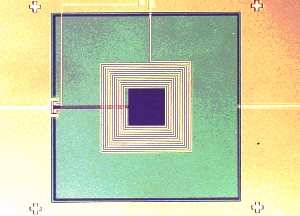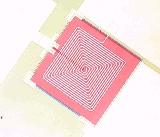Standard dc SQUIDs
Home

Publications

 dc SQUID "ESY - 10" L = 350 pH, Li = 1000 nH M = 20 nH, Ic = 8 mAdc SQUID "ESY - 20" L = 350 pH, Li = 600 nH M = 12 nH, Ic = 8 mAdc SQUID "ESY - 21" L = 350 pH, Li = 150 nH M = 5 nH, Ic = 8 mAdc SQUID "ESY - 22" L = 180 pH, Li = 280 nH M = 6 nH, Ic = 15 mAdc SQUID "ESY - 23" L = 75 pH, Li = 120 nH M = 3 nH, Ic = 25 mAL is the SQUID inductance, Li the inductance of the input coil, M the mutual inductance between SQUID and coil, and Ic the critical current of the SQUID.  Other values for M and Li are available on request.  The data listed above are typical values.  Some parameters are likely to vary slightly.

 ez SQUID Mess- und Analysegeräte Dr. Hans-Michael Mück, Herborner Strasse 9, 35764 Sinn, Germany email: info@ez-squid.de